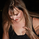6557 views
My Contribution to Jake Bernstein Educational Series, Initiated by Chris Moody.

The Stochastic Pop was developed by Jake Bernstein and modified by David Steckler. Bernstein's original Stochastic Pop is a trading strategy that identifies price pops when the Stochastic Oscillator surges above 80. Steckler modified this strategy by adding conditional filters using the Average Directional Index ( ADX ) and the weekly Stochastic Oscillator.

Modifications
1. Weekly Stochastic Oscillator for Trading Bias = 5* Daily Stochastic
2. Optional Volume Confirmation, Custom Average Volume Length

Future Plans
1. Adding Triggers for Entry, Stops and Target. - This will be release when we have ability to code the complete Strategy. Although it can be done with the current pinescript options, it would be far more easier if we have strategy ability .

http://stockcharts.com/school/doku.php?i...

-
UCSgears
```// Developed by Jake Bernstein
// Modified by David Steckler - Includes ADX Confirmation
// Modified by UCSgears - Optional Volume Confirmation
// Coded by UCSgears - Stochastic Pop and Drop Version 1

study(title="UCS_Stochastic Pop and Drop", shorttitle="UCS_JB SPOD", overlay = true)

// Input - Options
vlen = input(100, title="Volume Average Length")
vconf = input(true, title = "Volume Confirmation", type=bool)
steck = input(true, title = "David Steckler Modification", type=bool)
psl = input(true, title = "Plot Stop Loss", type = bool)

tbk = sma(stoch(close, high, low, 70), 3)
tbbull = tbk > 50
tbbear = tbk < 50

//Setup Definition
sdk = sma(stoch(close, high, low, 14), 3)
sdl = sdk > 80 and sdk < 80
sds = sdk < 20 and sdk > 20

up = change(high)
down = -change(low)
trur = rma(tr, 14)
plus = fixnan(100 * rma(up > down and up > 0 ? up : 0, 14) / trur)
minus = fixnan(100 * rma(down > up and down > 0 ? down : 0, 14) / trur)
sum = plus + minus
adx = 100 * rma(abs(plus - minus) / (sum == 0 ? 1 : sum), 14)

// Volume Confirmation
volma = sma(volume, vlen)
volconf = volume > volma

// Setup Long
sl = (steck and vconf) ? volconf == 1 and adxc == 1 and sdl == 1 and tbbull == 1 : (steck ==1 and vconf != 1) ? adxc == 1 and sdl == 1 and tbbull == 1 : (steck !=1 and vconf == 1) ? volconf == 1 and sdl == 1 and tbbull == 1 : sdl == 1 and tbbull == 1
// Setup Short
ss = (steck and vconf) ? volconf == 1 and adxc == 1 and sds == 1 and tbbear == 1 : (steck ==1 and vconf != 1) ? adxc == 1 and sds == 1 and tbbear == 1 : (steck !=1 and vconf == 1) ? volconf == 1 and sds == 1 and tbbear == 1 : sds == 1 and tbbear == 1

plotchar(sl, title="Long Setup", char='⇑', location=location.belowbar, color=green, transp=0, text="SPOD Long")
plotchar(ss, title="Short Setup", char='⇓', location=location.abovebar, color=red, transp=0, text="SPOD Short")

bc = sl == 1 ? green : ss == 1 ? red : na
barcolor(bc)
bgcolor(bc)

// Stop Loss
parsar = psl ? sar(0.02, 0.02, 0.2) : na
sc = psl and parsar < close ? green : red
plot(psl ? parsar : na, color=sc, linewidth = 2, style = circles)```